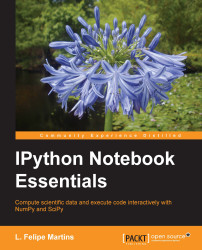•#### IPython Notebook Essentials#### Overview of this book

IPython Notebook EssentialsCreditswww.PacktPub.comPrefaceFree Chapter
A Tour of the IPython NotebookThe Notebook InterfaceGraphics with matplotlibHandling Data with pandasAdvanced Computing with SciPy, Numba, and NumbaProIPython Notebook Reference CardA Brief Review of PythonNumPy ArraysIndex## Overview of SciPy

`SciPy` is an extensive library for applied mathematics and scientific computation. The following is the complete list of all the modules available in the library:

Module

Functionality

`cluster`

Clustering algorithms

`constants`

Physical and mathematical constants

`fftpack`

Fast Fourier Transform

`integrate`

Integration and ordinary differential equations

`interpolate`

Interpolation and splines

`io`

Input and output

`linalg`

Linear algebra

`ndimage`

Image processing

`odr`

Orthogonal distance regression

`optimize`

Optimization and root-finding

`signal`

Signal processing

`sparse`

Sparse matrices

`spatial`

Spatial data structures

`special`

Special functions

`stats`

Statistical distributions

`weave`

C/C++ integration

The standard way to import `SciPy` modules in scripts is using the following command line:

```from scipy import signal
```

Then, individual functions can be called with the usual module reference syntax, as follows:

`signal.correlate(…)...`